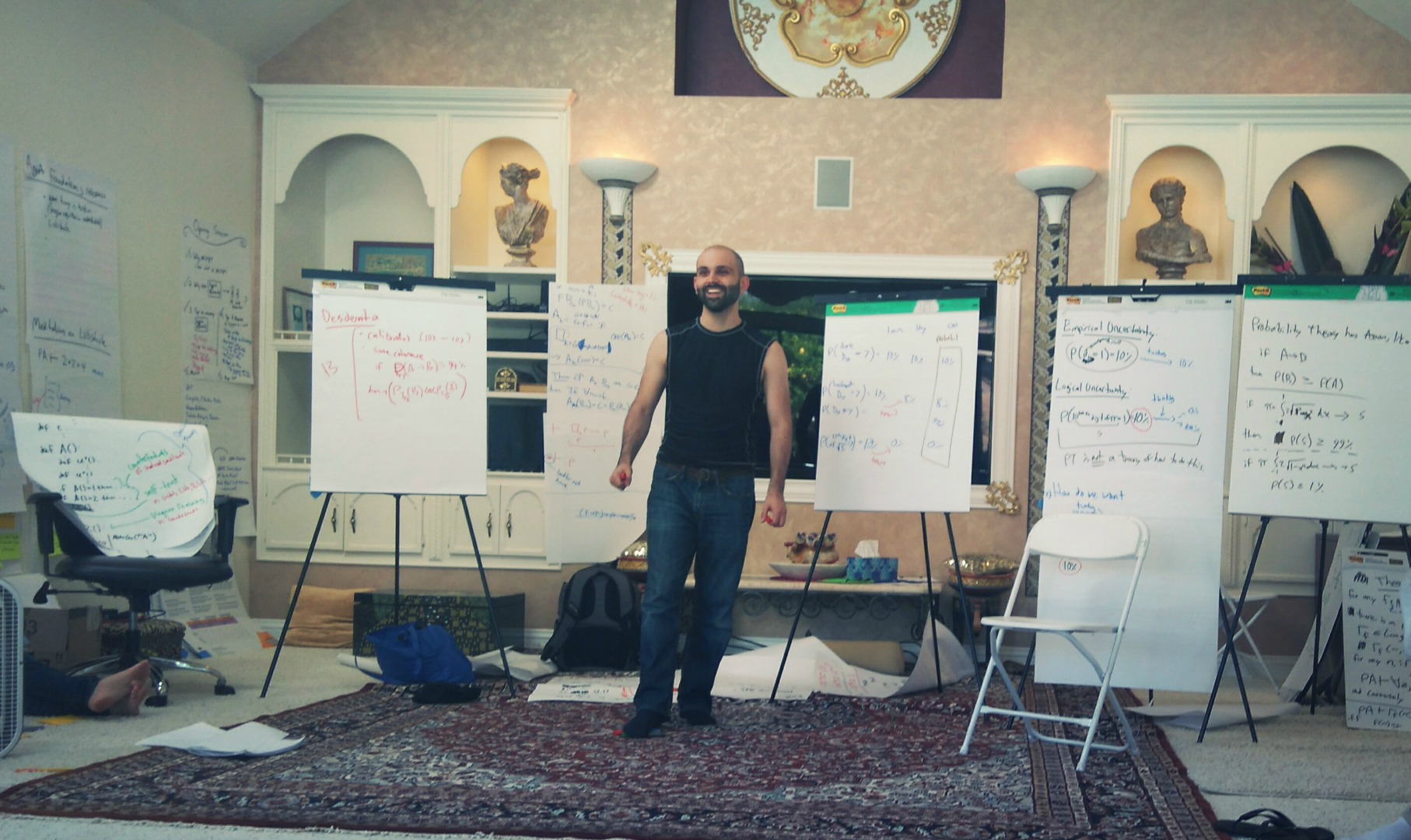# What's the probability of the Riemann Hypothesis?

Usually when we talk about probabilities, we have certain given information, which takes the form of a $$\sigma$$-algebra of possible events, and there is also a probability function that assigns values to each event. The rationality of a probability function is judged based on the relationships between events. For example if $$A \subseteq B$$ then we must have $$P(A) \leq P(B)$$. But as long as these relationships are satisfied (giving a proper probability measure), the probabilities could be anything. As such, we do not judge subjective probabilities based on whether they’re actually accurate or not, just whether they are consistent with each other.

Now, imagine if information isn’t the limiting factor in our uncertainty, but rather it’s our lack of mathematical knowledge. A statement like the Riemann Hypothesis (RH) is unknown even though it is entirely determined by the axiom system we use, leaving aside issues of completeness. Here there’s no given $$\sigma$$-algebra and in fact the relationships between RH and other statements may themselves be difficult to determine.

A more realistic view is that we have limited computational resources, we want to solve an intractible problem, and we’ll settle for the best approximation we can get. Thus a probability function is seen as a kind of approximation algorithm. With this algorithmic language, however, we aren’t able to give a very good answer for single propositions like RH. If RH is the entire set of inputs, the optimal approximation is the exact truth value, because it takes a trivial amount of computational resources to output the constant 1 or 0. If the set of inputs is infinite, then the particular input corresponding to RH makes no difference in an asymptotic analysis. For more elaboration on this theme, see this paper.

In traditional Bayesianism there is a seemingly ineradicable source of subjectivity from the choice of prefix Turing machine used to define Solomonoff’s prior. Any one input can be assigned a wide range of probabilities. Perhaps we are left with an analogous but different kind of subjectivity for mathematical probabilities.(Above: Andrew Critch thinking about this in Berkeley.)

* * *